# 《数据结构与算法分析》学习笔记-第十章-算法设计技巧

## 10.1 贪婪算法

### 10.1.1.1 单处理器

t1
t1+t2
t1+t2+t3
t1+t2+t3+t4



### 10.1.1.2 多处理器

CPU1 j1 j4 j7
CPU2 j2 j5 j8
CPU3 j3 j6 j9


### 10.1.2 Huffman编码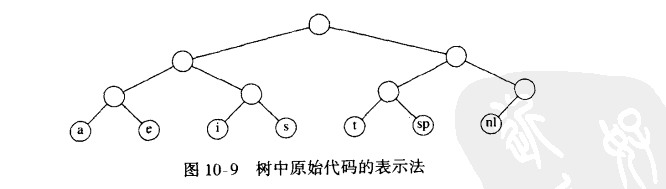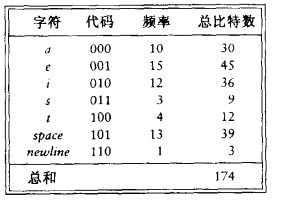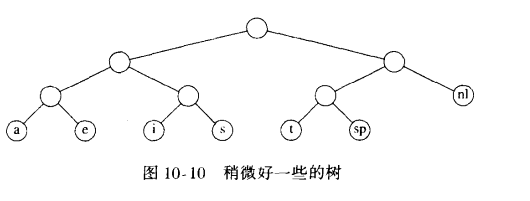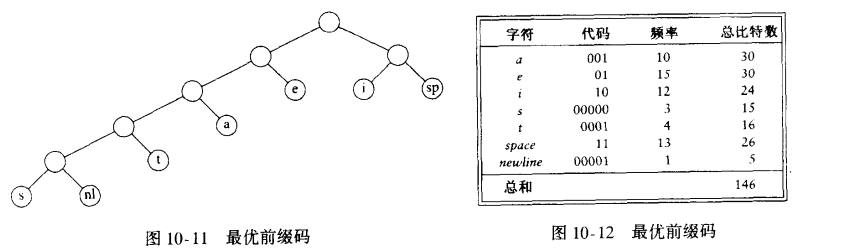#### 哈夫曼算法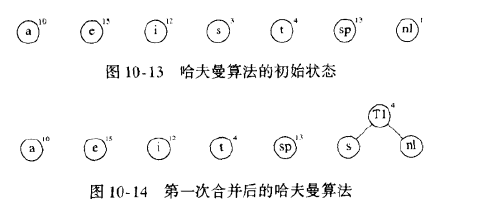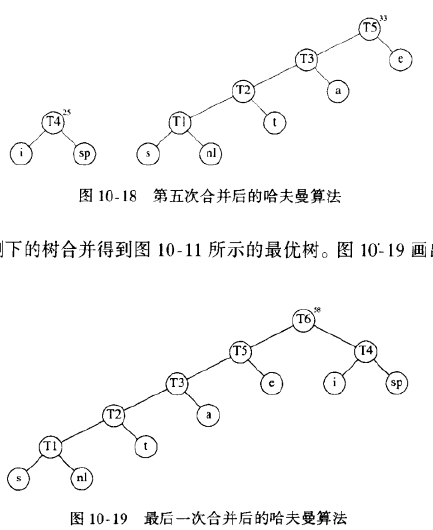1. 初始阶段，每个元素看成一棵单节点树。每个节点有自己的value和权重。
2. 将当前森林中，权值最低的两棵树进行合并，合并后新树的权值是老树权值的和
3. 继续进行第二步，不断将当前森林中权值最低的两棵树进行合并，合并时，左右分支任意，可以互换
4. 可以看出，权值（出现频率）越低，其深度越深；权值越高，其深度越浅。这样就能保证总开销最小。

### 10.1.3 近似装箱问题

1. 联机：必须解决当前问题，流程才能继续
2. 脱机：必须了解完所有的问题，流程才能开始

#### 10.1.3.1 联机算法

• 定理：存在使得任意联机装箱算法至少使用4/3最优箱子数的输入
##### 1. 下项适合算法

• 令M是将一列物品I装箱所需的最优装箱数，则下项适合算法所用箱数绝不超过2M个箱子。存在一些顺序使得下项适合算法用箱2M-2个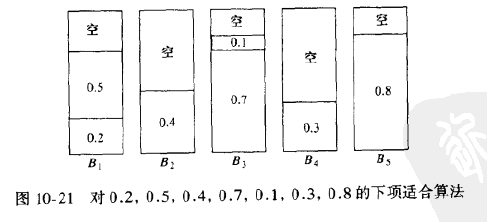##### 2. 首次适合算法##### 3. 最佳适合算法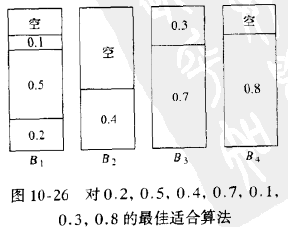#### 10.1.3.2 脱机算法

• 令N项物品的输入大小（以递减顺序排序）分别为s1, s2, ... , sN。并设最优装箱方法使用M个箱子。那么，首次适合递减算法放到外加的箱子中的所有物品的大小最多为1/3
• 放入外加的箱子中的物品的个数最多是M-1
• 令M时物品集I装箱所需的最优箱子数，则首次适合递减算法所用箱子数绝不超过(4M+1)/3
• 令M是将物品集I装箱所需要的最优箱子数，则首次适合递减算法所用箱子数绝不超过11/9 * M + 4。存在使得首次适合递减算法用到11/9 * M个箱子的序列

## 10.2 分治算法

• 分：递归解决较小的问题（基本情况除外）
• 治：从子问题的解，构建原问题的解

### 10.2.1 分治算法的运行时间

方程T(N)=aT(N/b)+Θ(N^k)的解为
T(N)=
O(N^(log(b)a)), 若a>b^k
O(N^k * logN), 若a=b^k
O(N^k), 若a<b^k

T(N)=
O(N^(log(b)a)), 若a>b^k
O(N^k * (logN)^(p+1)), 若a=b^k
O(N^k * (logN)^p), 若a<b^k
a >=1, b> 1 且p >=0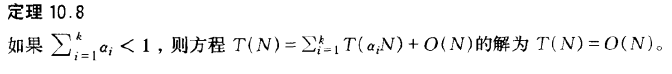### 10.2.2 最近点问题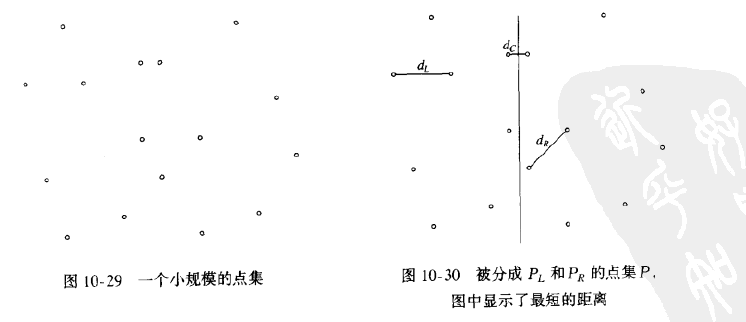1. 蛮力计算
for(i = 0; i<NumPointsInStrip; i++)
for(j=i+1; j<NumPointsInStrip; j++)
if(Distance(Pi, Pj) < x)
x = Distance(Pi,Pj);

1. 精炼计算
for(i = 0; i<NumPointsInStrip; i++)
for(j=i+1; j<NumPointsInStrip; j++)
if (Pi和Pj的y坐标相差大于x)
break;
else
if(Distance(Pi, Pj) < x)
x = Distance(Pi,Pj);


### 10.2.3 选择问题

1. 把N个元素分成[N/5]组，5个元素一组，忽略（最多4个）剩余的元素
2. 找出每组的中项，得到[N/5]个中项的表M
3. 求出M的中项，将其作为枢纽元V返回

### 10.2.4 一些运算问题的理论改进

#### 10.2.4.1 整数相乘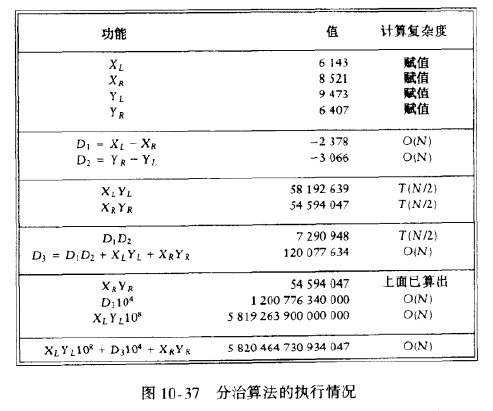#### 10.2.4.2 矩阵乘法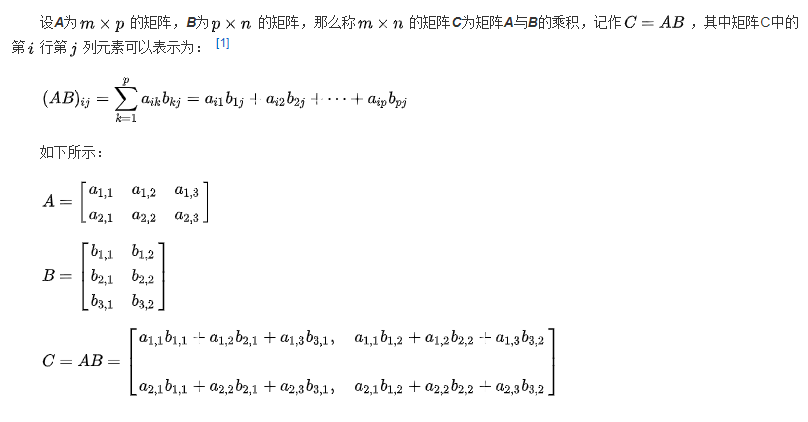1. 当矩阵A的列数（column）等于矩阵B的行数（row）时，A与B可以相乘。
2. 矩阵C的行数等于矩阵A的行数，C的列数等于B的列数。
3. 乘积C的第m行第n列的元素等于矩阵A的第m行的元素与矩阵B的第n列对应元素乘积之和。

void
MatrixMultiply(Matrix A, Matrix B, Matrix C, int N)
{
int i, j, k;

for (i = 0; i < N; i++)
for (j = 0; j < N; j++)
C[i][j] = 0;

for (i = 0; i < N; i++)
for (j = 0; j < N; j++)
for (k = 0; k < N; k++)
C[i][j] += A[i][k] * B[k][j];
}


## 10.3 动态规划

### 10.3.1 用一个表代替递归

1. 斐波那契数的低效算法
int
Fib(int N)
{
if (N <= 1)
return 1;
else
return Fib(N - 1) + Fib(N - 2);
}


1. 斐波那契数的线性算法
int
Fibonacci(int N)
{

if (N <= 1)
return 1;

Last = NextToLast = 1;
for (i = 2; i <= N; i++)
{
NextToLast = Last;
}

}


### 10.3.2 矩阵乘法的顺序安排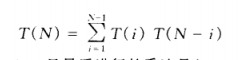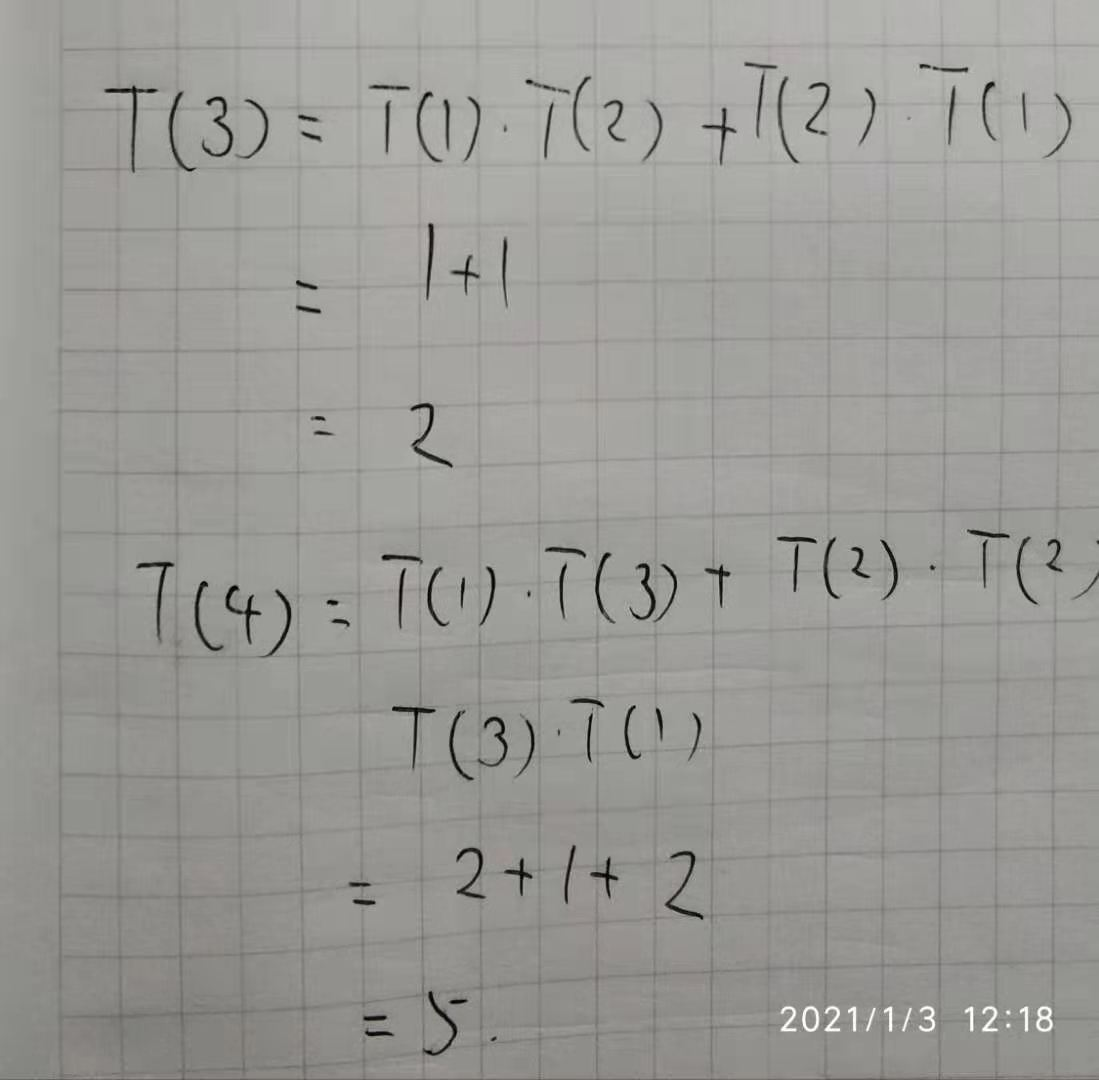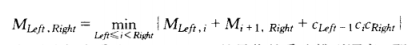找出矩阵乘法最优顺序的程序
void
OptMatrix(const long C[], int N, TwoDimArray M, TwoDimArray LastChange)
{
int i, k, Left, Right;
long ThisM;

for (Left = 1; Left <=N; Left++)
M[Left][Left] = 0;
for (k = 1; k < N; k++)
for (Left = 1; Left <= N-k; Left++)
{
/* for each position */
Right = Left + k;
M[Left][Right] = Infinity;
for (i = Left; i < Right; i++)
{
ThisM = M[Left][i] + M[i+1][Right] + C[Left - 1]*C[i]*C[Right];
if (ThisM < M[Left][Right])
{
M[Left][Right] = ThisM;
LastChange[Left][Right] = i;
}
}
}
}


### 10.3.3 最优二叉查找树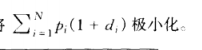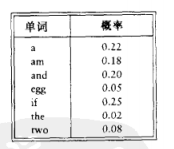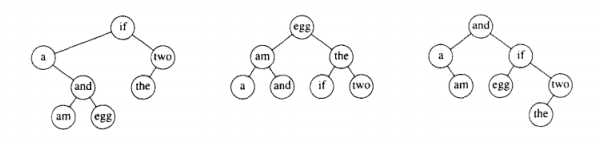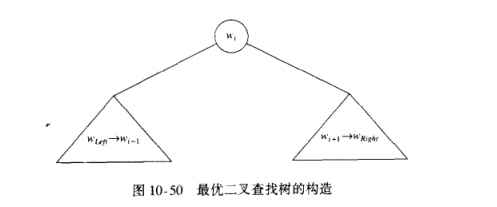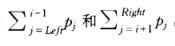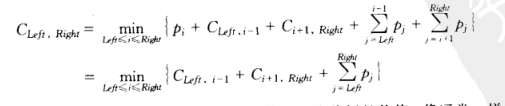### 10.3.4 所有点对最短路径

void
AllPairs(TwoDimArray A, TwoDimArrayD, TwoDimArray Path, int N)
{
int i, j, k;

/* Initialize D and Path */
for (i = 0; i < N; i++)
for (j = 0; j < N; j++)
{
D[i][j] = A[i][j];
Path[i][j] = NotAVertex;
}

for (k = 0; k < N; k++)
/* Consider each vertex as an intermediate */
for (i = 0; i < N; i++)
for (j = 0; j < N; j++)
if (D[i][k] + D[k][j] < D[i][j])
{
/* Update shortest path */
D[i][j] = D[i][k] + D[k][j];
Path[i][k] = k;
}
}


## 参考文献

1. Mark Allen Weiss.数据结构与算法分析[M].America, 2007

posted @ 2021-02-20 23:29  CrazyCatJack  阅读(429)  评论(0编辑  收藏  举报